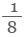The release of radiation by unstable nuclei is called radioactive decay. This process occurs naturally and cannot be influenced by chemical or physical processes. The release of radiation is also a random event and overtime the activity of the radioactive material decreases. It is not possible to predict when an individual nucleus in a radioactive material will decay. But it is possible to measure the time taken for half of the nuclei in a radioactive material to decay. This is called the half life of radioactive material or radioisotope.

Half life can be defined as the time taken for the number of nuclei in a radioactive material to halve. It can also be defined as the time taken for the count rate of a sample of radioactive material to fall to half of its starting level.

The count rate is measured by using an instrument called a Geiger-Muller tube over a period of time. A Geiger-Muller tube detects radiations by absorbing the radiation and converting it into an electrical pulse which triggers a counter and is displayed as a count rate. [How Geiger-Muller tube works?]

Radioactive elements have a wide range of half life values.  The isotope Uranium-238 has a half life as long as 4.5 billion years whereas the half life of Thorium-234 is as little as 24 days.

The animation below explains the half life of a radioactive isotope.

At the start of the measurement the radioisotope has 10,000 unstable nuclei. Over 2 days 5,000 of these unstable nuclei undergo radioactive decay to stable nuclei. Therefore half of the original radioactive nuclei have decayed in 2 days so the half life for this radioisotope is 2 days.

Over the next 2 days 2500 of the remaining radioactive nuclei undergo decay. This is half of the remaining 5000 radioactive nuclei.

The isotope undergoes further decay and it takes 2 days for the number of radioactive nuclei to halve in number.

The half life for this radioisotope is 2 days. Therefore the number of radioactive nuclei is always half the number there was 2 days ago. The curve produced is called a radioactive decay curve.

### Calculating Half Life

We know that after one half life, one half of the original radioactive nuclei remain. After two half lives, one half of this half remains or one quarter of the original radioactive nuclei remain.

We can therefore say:

1 half life          =           ½ of the original radioactive nuclei remain
2 half lives       =          ¼ of the original radioactive nuclei remain

This can be written as an equation as:### Example:

A radioactive isotope has a radioactivity measured on a Geiger-Muller tube at 10,000 Becquerel’s or 10,000 counts per second. What is the activity of the radioisotope after 3 half lives?

The amount remaining =x Original amount

The amount remaining =x 10,000

The amount remaining =x 10,000

The amount remaining = 1250 Bq

For this question we did not need to know the half life of the isotope, we simply needed to remember the equation and the fact that the activity is halved for every half life.